# US7379831B1 - Error correction in an oversampled ADC using few stored calibration coefficients - Google Patents

## Info

Publication number
US7379831B1
US7379831B1 US11/472,192 US47219206A US7379831B1 US 7379831 B1 US7379831 B1 US 7379831B1 US 47219206 A US47219206 A US 47219206A US 7379831 B1 US7379831 B1 US 7379831B1
Authority
US
United States
Prior art keywords
uncorrected
transfer function
digital values
non
volatile memory
Prior art date
Legal status (The legal status is an assumption and is not a legal conclusion. Google has not performed a legal analysis and makes no representation as to the accuracy of the status listed.)
Active
Application number
US11/472,192
Inventor
Anatoliy V. Tsyrganovich
Current Assignee (The listed assignees may be inaccurate. Google has not performed a legal analysis and makes no representation or warranty as to the accuracy of the list.)
IXYS Intl Ltd
Original Assignee
Priority date (The priority date is an assumption and is not a legal conclusion. Google has not performed a legal analysis and makes no representation as to the accuracy of the date listed.)
Filing date
Publication date
Priority to US10/844,901 priority Critical patent/US6993441B1/en
Priority to US11/267,722 priority patent/US7085663B1/en
Priority to US11/472,192 priority patent/US7379831B1/en
Assigned to ZILOG, INC. reassignment ZILOG, INC. ASSIGNMENT OF ASSIGNORS INTEREST (SEE DOCUMENT FOR DETAILS). Assignors: TSYRGANOVICH, ANATOLIY V.
Application granted granted Critical
Publication of US7379831B1 publication Critical patent/US7379831B1/en
Assigned to IXYS CH GMBH reassignment IXYS CH GMBH ASSIGNMENT OF ASSIGNORS INTEREST (SEE DOCUMENT FOR DETAILS). Assignors: ZILOG, INC.
Assigned to IXYS INTL LIMITED reassignment IXYS INTL LIMITED ASSIGNMENT OF ASSIGNORS INTEREST (SEE DOCUMENT FOR DETAILS). Assignors: IXYS CH GMBH
Application status is Active legal-status Critical
Anticipated expiration legal-status Critical

• 230000015654 memory Effects 0 abstract claims description 44
• 230000000875 corresponding Effects 0 abstract claims description 21
• 238000005365 production Methods 0 claims description 2
• 229920002574 CR-39 Polymers 0 title 1
• 238000004458 analytical methods Methods 0 description 14
• 239000004676 acrylonitrile butadiene styrene Substances 0 description 8
• 230000035969 Vmax Effects 0 description 5
• 230000001965 increased Effects 0 description 5
• 230000001747 exhibited Effects 0 description 3
• 230000003044 adaptive Effects 0 description 2
• 238000004364 calculation methods Methods 0 description 2
• 238000009499 grossing Methods 0 description 2
• 239000011133 lead Substances 0 description 2
• 230000032683 aging Effects 0 description 1
• 230000001721 combination Effects 0 description 1
• 238000009826 distribution Methods 0 description 1
• 230000002708 enhancing Effects 0 description 1
• 238000001914 filtration Methods 0 description 1
• 230000004301 light adaptation Effects 0 description 1
• 238000006011 modification Methods 0 description 1
• 230000004048 modification Effects 0 description 1
• 230000004044 response Effects 0 description 1
• 230000000717 retained Effects 0 description 1
• 238000005070 sampling Methods 0 description 1
• 230000011218 segmentation Effects 0 description 1

## Images

•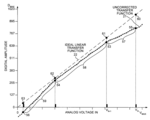••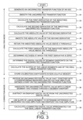•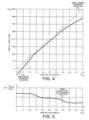••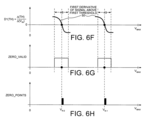•••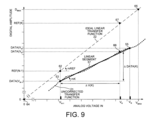•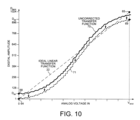••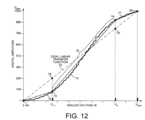••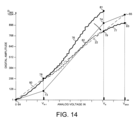••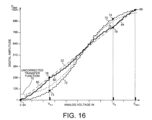•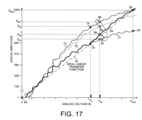## Classifications

• HELECTRICITY
• H03BASIC ELECTRONIC CIRCUITRY
• H03MCODING; DECODING; CODE CONVERSION IN GENERAL
• H03M3/00Conversion of analogue values to or from differential modulation
• H03M3/30Delta-sigma modulation
• H03M3/38Calibration
• H03M3/386Calibration over the full range of the converter, e.g. for correcting differential non-linearity
• H03M3/388Calibration over the full range of the converter, e.g. for correcting differential non-linearity by storing corrected or correction values in one or more digital look-up tables
• HELECTRICITY
• H03BASIC ELECTRONIC CIRCUITRY
• H03MCODING; DECODING; CODE CONVERSION IN GENERAL
• H03M3/00Conversion of analogue values to or from differential modulation
• H03M3/30Delta-sigma modulation
• H03M3/458Analogue/digital converters using delta-sigma modulation as an intermediate step

## Abstract

An analog-to-digital converter (ADC) exhibiting an uncorrected non-linear transfer function receives measured analog voltage amplitudes and outputs uncorrected digital values. A calibration circuit receives each uncorrected digital value and outputs a corrected digital value. The measured analog voltage amplitudes received by the ADC and the corresponding corrected digital values output by the calibration circuit define points approximating an ideal linear transfer function of the ADC. The calibration circuit performs piecewise-linear approximation of the uncorrected transfer function and associates each uncorrected digital value with one of N linear segments that join at inflection points on the uncorrected transfer function. The inflection points are determined using the second derivative of the uncorrected transfer function. The calibration circuit calculates each corrected digital value using no more than 2N+2 stored calibration coefficients. Calibration coefficients that are not stored in non-volatile memory are calculated upon power up using stored calibration coefficients.

## Description

CROSS REFERENCE TO RELATED APPLICATION

This application is a continuation-in-part of, and claims priority under 35 U.S.C. §120 from, nonprovisional U.S. patent application Ser. No. 11/267,722 entitled “Adaptive Error Correction in an Oversampled ADC,” now U.S. Pat. No. 7,085,663, issued Aug. 1, 2006, filed on Nov. 4, 2005, the subject matter of which is incorporated herein by reference application Ser. No. 11/267,722, in turn, is a continuation of U.S. patent application Ser. No. 10/844,901 entitled “Adaptive Error Correction in an Oversampled ADC,” now U.S. Pat. No. 6,993,441, filed on May 12, 2004, the subject matter of which is incorporated herein by reference.

TECHNICAL FIELD

The present invention relates to analog-to-digital converters, and more specifically to a memory-saving method of calibrating analog-to-digital converters.

BACKGROUND

Analog-to-digital converters (ADCs) are widely used to convert analog voltage signals into digital output signals. ADCs are often embedded with other components in integrated circuits, for example, in microcontrollers. The performance of. ADCs that are embedded in integrated circuits is often worse than the performance of standalone ADCs with the same specification. The presence of noise on signal lines and power supply lines often results in higher signal interference and output errors in embedded ADCs. Another source of errors in ADCs is the nonlinearity of the transfer functions of the ADCs. Thus, calibrating embedded ADCs to correct for errors can significantly enhance performance.

There are various known methods for calibrating ADCs to correct for gain error, dc offset error, INL error and DNL error. In one method, the uncorrected digital output of an ADC with a nonlinear transfer function is mapped to the ideal linear transfer function. The calibration method determines a correction factor for each uncorrected digital value output by the ADC and stores the uncorrected value and its associated correction factor in a lookup table. A calibration circuit outputs a corrected digital value on the ideal linear transfer function by adding the appropriate correction factor to each uncorrected digital value output by the ADC. Valuable memory resources must be allocated to the lookup table. A calibration method that consumes less memory resources for a lookup table would be particularly advantageous in an ADC that is embedded in a microcontroller.

Another calibration method involves piecewise linear segmentation. The entire dynamic range of the uncorrected output of an ADC is divided into equally-spaced ranges of digital values. A best-fitting linear segment is then mapped to the distribution of digital values within each range. Each uncorrected digital output value is associated with a point on a linear segment. Correction factors are then calculated for each point along each linear segment. The output of the ADC is corrected by adding to each uncorrected digital value the correction factor associated with the closest point on the appropriate linear segment.

A calibration method that divides an uncorrected nonlinear transfer function into segments of approximately equal length is less accurate for segments where the uncorrected transfer function is most nonlinear. Moreover, depending on the shape of the uncorrected transfer function, calculating correction factors with additional segments does not necessarily render the calibration more accurate. For an uncorrected, hockey-stick-shaped transfer function, for example, approximating the uncorrected transfer function with successive linear segments along the handle does not provide a better fit than would a single linear segment. Calculating correction factors for the successive linear segments does not significantly improve the resolution of the ADC.

A method is sought for calibrating an analog-to-digital converter that reduces the memory used to store correction factors and that also reduces any calculations that do not significantly improve the resolution of the analog-to-digital converter.

SUMMARY

An analog-to-digital converter (ADC) that exhibits an uncorrected non-linear transfer function receives measured analog voltage amplitudes and outputs uncorrected digital values. A calibration circuit receives each uncorrected digital value and outputs a corrected digital value. The calibration circuit performs piecewise-linear approximation of the uncorrected non-linear transfer function and associates each uncorrected digital value with the applicable linear segment that passes through a segment endpoint on the uncorrected transfer function. The corrected digital values output by the calibration circuit and the corresponding measured analog voltage amplitudes received by the ADC define points approximating an ideal linear transfer function of the ADC. In one embodiment, the calibration circuit is the processing unit of a microcontroller.

The calibration circuit calculates each corrected digital value using calibration coefficients associated with the applicable linear segment. One calibration coefficient is the endpoint digital value of a segment endpoint of each linear segment. Another calibration coefficient is the slope of each linear segment. The calibration circuit determines the calibration coefficients by calculating the second derivative of the uncorrected transfer function. The segment endpoint of each linear segment is located on the uncorrected transfer function at a local maximum of a second derivative of the uncorrected transfer function.

The calibration coefficients are stored in non-volatile memory. The corrected digital values are generated using three calibration coefficients plus four additional calibration coefficients for each segment of the piecewise-linear approximation of the uncorrected transfer function. Thus, the calibration circuit can generate the corrected digital values using fewer calibration coefficients than the predetermined number of digital values into which the ADC converts the measured analog voltage amplitudes.

A method calibrates an ADC having a non-linear transfer function by receiving a plurality of uncorrected digital values from the ADC and by outputting a plurality of corrected digital values that approximately track the ideal linear transfer function of the ADC. The method approximates the non-linear transfer function with linear segments. A segment endpoint of each linear segment is located on the non-linear transfer function at a local maximum of the second derivative of the non-linear transfer function. The method calculates a corrected digital value for each uncorrected digital value using calibration coefficients associated with the linear segment applicable to the uncorrected digital value. The calibration coefficients include the endpoint digital values of segment endpoints of each linear segment. The method stores the calibration coefficients in non-volatile memory. In one embodiment, corrected digital values are not generated by adding a separate, stored correction factor to each uncorrected digital value.

In another embodiment, the corrected digital values are generated using no more than two stored calibration coefficients plus two additional stored calibration coefficients for each segment of the piecewise-linear approximation of the uncorrected transfer function. Additional calibration coefficients are calculated during operation using the calibration coefficients stored in non-volatile memory. In this manner, the calibration circuit can generate the corrected digital values using relatively few stored calibration coefficients. Thus, space in non-volatile memory is conserved. In yet another embodiment, the corrected digital values are generated using no more than two stored calibration coefficients for each segment of the piecewise-linear approximation of the uncorrected transfer function.

Other embodiments and advantages are described in the detailed description below. This summary does not purport to define the invention. The invention is defined by the claims.

BRIEF DESCRIPTION OF THE DRAWINGS

The accompanying drawings, where like numerals indicate like components, illustrate embodiments of the invention.

FIG. 1 is a simplified schematic block diagram of a calibration circuit and an analog-to-digital (ADC) converter embedded in a microcontroller.

FIG. 2 is a graph of an uncorrected, nonlinear transfer function of the ADC of FIG. 1.

FIG. 3 is a flowchart of steps whereby the calibration circuit of FIG. 1 calibrates the ADC of FIG. 1.

FIG. 4 is a graph of the transfer function of FIG. 2 after being smoothed.

FIG. 5 is a graph of the first derivative of the transfer function of FIG. 4.

FIGS. 6A-H are graphs of derivative and absolute value functions of the first derivative function of FIG. 5.

FIG. 7 shows three graphs correlating input analog voltage amplitudes to corresponding inflection points on the first derivative of FIG. 4 and the uncorrected transfer function of FIG. 1.

FIG. 8 illustrates the uncorrected transfer function of FIG. 1 being approximated by three linear segments.

FIG. 9 illustrates the calculation of the slope of one linear segment of FIG. 8.

FIG. 10 is a graph of an uncorrected transfer function that is shifted by an additional embodiment of the calibration circuit of FIG. 1.

FIG. 11 is a graph of a shifted and gain-corrected transfer function generated by the additional embodiment of the calibration circuit of FIG. 1.

FIG. 12 illustrates the shifted and gain-corrected transfer function of FIG. 11 being approximated by three linear segments.

FIG. 13 illustrates offset and gain correction performed on the three linear segments of FIG. 12 by the additional embodiment of the calibration circuit.

FIG. 14 illustrates gain correction performed on the second linear segment of FIG. 12 by the additional embodiment of the calibration circuit.

FIG. 15 illustrates gain correction performed on the third linear segment of FIG. 12 by the additional embodiment of the calibration circuit.

FIG. 16 is a graph of the uncorrected transfer function of FIG. 10, the shifted and gain-corrected transfer function of FIG. 11, and the corrected transfer function that includes the three corrected portions shown in FIGS. 13-15.

FIG. 17 illustrates the correction performed by the additional embodiment of the calibration circuit on an uncorrected digital value to generate a corrected digital value using few stored calibration coefficients.

DETAILED DESCRIPTION

Reference will now be made in detail to some embodiments of the invention, examples of which are illustrated in the accompanying drawings.

FIG. 1 shows microcontroller integrated circuit 10 with a calibration circuit 11, an embedded sigma-delta analog-to-digital converter (ADC) 12, and a non-volatile memory 13. Calibration circuit 11 has an input bus 14 and an output bus 15. ADC 12 has an input lead 16 and an output bus 17. ADC 12 receives a continuous analog input signal on input lead 16. The continuous analog input signal comprises a plurality of measured analog voltage amplitudes 18.

ADC 12 oversamples the continuous analog input signal at a sampling frequency that is at least twice as great as the frequency of the analog input signal. Oversampling spreads quantization noise over a wide frequency range. ADC 12 converts the plurality of measured analog voltage amplitudes 18 into a corresponding plurality of uncorrected digital values 19. Each of the plurality of measured analog voltage amplitudes 18 is ideally converted into one of a predetermined number of possible digital values. In this embodiment, measured analog voltage amplitudes 18 are ideally converted into 1024 (210) possible 10-bit digital values. Each uncorrected digital value 19, however, has twelve bits so that those uncorrected digital values that fall outside the 1024 possible 10-bit digital states can be represented. Each uncorrected digital value 19 has ten regular bits, a sign bit, and an overflow bit. ADC 12 outputs the plurality of 12-bit uncorrected digital values 19 onto 12-bit output bus 17.

Each measured analog voltage amplitude and the corresponding uncorrected digital value define a point on an uncorrected transfer function of ADC 12. The uncorrected transfer function of ADC 12 is not linear. The plurality of uncorrected digital values 19 exhibits gain error, dc offset error, INL error and DNL error. ADC 12 would ideally convert linearly increasing analog voltage amplitudes into linearly increasing digital values, resulting in an ideal linear transfer function.

Calibration circuit 11 receives the plurality of uncorrected digital-values 19 onto input bus 14 and outputs a plurality of corrected digital values 20 onto output bus 15. Calibration circuit 11 corrects for total error such that the plurality of measured analog voltage amplitudes 18 received by ADC 12 and the corresponding plurality of corrected digital values 20 output by calibration circuit 11 define points approximating the ideal linear transfer function of ADC 12. Thus, calibration circuit 11 does not separately correct for gain error, dc offset error, INL error and DNL error. Calibration circuit 11 generates the corrected digital values 20 using calibration coefficients that correspond to linear segments that approximate the plurality of uncorrected digital values 19. For each measured analog voltage amplitude received by ADC 12, calibration circuit 11 is capable of calculating a corrected digital value to within +/− one half of the least significant bit (LSB) of the digital value that corresponds to the measured analog voltage amplitude on the ideal linear transfer function.

FIG. 2 is a graph of a hypothetical, uncorrected transfer function 21 of ADC 12, as well as an ideal linear transfer function 22 for ADC 12. Uncorrected transfer function 21 correlates physically measured analog voltage amplitudes received by ADC 12 to uncorrected digital values output by ADC 12. ADC 12 outputs the uncorrected digital values as 12-bit digital values. FIG. 2 shows that DC offset error causes a portion of uncorrected transfer function 21 to extend into negative digital amplitudes. The uncorrected digital values 19 in this negative portion of uncorrected transfer function 21 are output by ADC 12 as negatively signed digital numbers.

FIG. 3 is a flowchart illustrating steps 23-39 of a method by which calibration circuit 11 receives the plurality of uncorrected digital values 19 and outputs the plurality of corrected digital values 20. Calibration circuit 11 thereby calibrates uncorrected, nonlinear transfer function 21 of ADC 12 by outputting the plurality of corrected digital values 20 that approximately track ideal linear transfer function 22. In a first step 23, ADC 12 generates uncorrected transfer function 21. Each point on uncorrected transfer function 21 is defined by a measured analog voltage amplitude and a corresponding uncorrected digital value. In an analysis mode of microcontroller 10, uncorrected transfer function 21 is analyzed. The analysis mode is, for example, performed during testing after production. Subsequent steps of FIG. 3 are now described in relation to the functions shown in the graphs of FIGS. 4-9.

The analysis mode consists of steps 2′ through 34, in which inflection points on uncorrected transfer function 21 are determined. Uncorrected transfer function 21 is then approximated as multiple piecewise-linear segments, wherein the linear segments join at the inflection points of uncorrected transfer function 21. The number of linear segments is one more than the number of inflection points, unlike in other calibration methods where the number of linear segments is defined irrespective of characteristics of the uncorrected transfer function.

In a step 24, uncorrected transfer function 21 is smoothed by filtering in test equipment. FIG. 4 shows the resulting smoothed, uncorrected transfer function 40. Step 24 is not essential to the method of FIG. 3. The smoothing of uncorrected transfer function 21 reduces the number of closely-spaced inflection points and, therefore, prevents short linear segments to be used in the approximation of uncorrected transfer function 21. Smoothing reduces the number of calibration coefficients used in the method of FIG. 3, but also reduces the resolution of the calibration achieved by calibration circuit 11.

In a step 25, the first derivative of the smoothed, uncorrected transfer function 40 is determined. FIG. 5 is a graph of the first derivative (D1) 41 of smoothed, uncorrected transfer function 40. First derivative (D1) 41 depicts the change of uncorrected digital values of smoothed transfer function 40 in relation to the change in the measured input analog voltage amplitude.

In a step 26, the second derivative of smoothed, uncorrected transfer function 40 is calculated. FIG. 6B shows the second derivative (D2) 42 of the smoothed, uncorrected transfer function 40. Second derivative (D2) 42 depicts the change of first derivative (D1) 41 in relation to the change in the measured input analog voltage amplitude.

In a step 27, the absolute value of second derivative 42 of smoothed, uncorrected transfer function 40 is calculated. FIG. 6C shows the absolute value (D2 ABS) 43 of second derivative (D2) 42.

In a step 28, absolute value (D2 ABS) 43 of second derivative (D2) 42 is filtered to generate a smoothed function. FIG. 6D shows the smoothed absolute value F(D2 ABS) 44 of second derivative (D2) 42.

In a step 29, points on smoothed absolute value F(D2 ABS) 44 are discarded for amplitude values that fall below a first threshold 45. First threshold 45 is placed at an amplitude above the noise level of absolute value (D2 ABS) 43 and smoothed absolute value F(D2 ABS) 44. By lowering threshold 45 to an amplitude just above the noise level, the piecewise-linear segments approximate uncorrected transfer function 21 to a resolution of less than +/−½ LSB. Therefore, calibration circuit 11 can correct for DNL error that is greater than +/−½ LSB. DNL error of greater than +/−½ LSB would result in one bit of effective resolution being lost. Further increases in DNL error would cause a missing code phenomenon.

FIG. 6E shows the portions (TH) 46 of smoothed absolute value F(D2 ABS) 44 that are retained above first threshold 45. A higher second threshold 47 is then applied to the portions (TH) 46 in order to define valid ranges of input analog voltage in which inflection points on uncorrected transfer function 21 can be located. Two valid ranges 48-49 of input analog voltage are shown in FIG. 6E.

In a step 30, the first derivative of the portions (TH) 46 of smoothed absolute value F(D2 ABS) 44 are calculated. FIG. 6F shows the first derivative D1(TH) 50 of the portions (TH) 46. First derivative D1(TH) 50 depicts the change of portions (TH) 46 in relation to the change in the measured input analog voltage amplitude. A zero crossing signal is generated at the points where first derivative D1(TH) 50 crosses zero. A zero valid signal (ZERO_VALID) is generated that indicates the zero-crossing points of the zero crossing signal that fall within the valid ranges 48-49 determined with second threshold 47.

In a step 31, the measured input analog voltage amplitudes are determined for the valid zero-crossing points. FIG. 6H shows a ZERO_POINTS signal indicating two input analog voltage amplitudes VN−1 and VN−2 corresponding to inflection points on uncorrected transfer function 21.

In a step 32, the digital values of the inflection points on uncorrected transfer function 21 that correspond to the valid zero-crossing points are determined. FIG. 7 illustrates that the two input analog voltage amplitudes VN−1 and VN−2 correspond to inflection points 51-52 on first derivative (D1) 41 and to inflection points 53-54 on uncorrected transfer function 21. Inflection points 53-54 represent points at which uncorrected transfer function 21 changes its slope by an amount greater than the slope changes indicated by local maxima of function 43 below first threshold 45 in FIG. 6C. The digital values DATA(VN−1) and DATA(VN−2) of the inflection points 53-54 on uncorrected transfer function 21 are then determined empirically in the analysis mode.

End points 55-56 on uncorrected transfer function 21 are then empirically chosen that correspond to the ends of the dynamic range of ADC 12. Inflection points 53-54 and end points 55-56 are defined to be segment endpoints on uncorrected transfer function 21. Uncorrected transfer function 21 is then approximated by three linear segments that connect the two inflection points 53-54 and the end points 55-56.

FIG. 8 shows that uncorrected transfer function 21 is approximated by three linear segments 57-59. Linear segment 57 joins end point 55 and inflection point 53; linear segment 58 joins inflection point 53 and inflection point 54; and linear segment 59 joins inflection point 54 and end point 56. Uncorrected transfer function 21 is a relatively smooth function and is accurately approximated with just three linear segments. The method of FIG. 3 approximates uncorrected transfer function 21 with fewer linear segments than would have resulted from a conventional piecewise-linear approximation that inserts a linear segment for each predetermined range of digital values. For example, where a conventional piecewise-linear approximation inserts a linear segment for each range of two hundred digital values, uncorrected transfer function 21 would be approximated with about five linear segments. An approximation using five segments over equal digital ranges, as opposed to the three segments generated by the method of FIG. 3, would involve more calculations but would nevertheless result in poorer resolution. The method of FIG. 3 places the endpoints of the linear segments only at inflection points of uncorrected transfer function 21 and thereby concentrates the linear segments in curvy areas of the uncorrected transfer function, which both reduces the number of calibration coefficients and improves resolution.

In a step 33, the slopes of ideal linear transfer function 22 and of the linear segments 57-59 are calculated. FIG. 9 shows a portion of the graph of FIG. 8 and illustrates the calculation of the slope of ideal linear transfer function 22 and the slope of linear segment 57. Still in the analysis mode, the end points of ideal linear transfer function 22 are empirically determined. Ideal linear transfer function 22 extends from point 64 (VMIN, DMIN) to point 65 (VMAX, DMAX). In this example, VMIN is zero volts and DMIN is digital zero; VMAX is five volts and DMAX is 1023. The slope (mREF) of ideal linear transfer function 22 is (DMAX−DMIN)/(VMAX−VMIN) or, in this example, 1023/5 volts. End point 53 of linear segment 57 has the coordinates (VN−1, DATA(VN−1)). End point 55 has the coordinates (VN, DATA(VN)). Thus, the slope (mK) of linear segment 57 is [DATA(VN)−DATA(VN−1)]/(VN−VN−1).

In step 33, the digital values of points 60-63 on ideal linear transfer function 22 are also determined empirically. Points 60-63 have the same x-coordinates (analog voltage) as do end point 55, inflection points 53-54 and end points 56, respectively. For example, the digital value of point 62 with an x-coordinate of VN−1 is empirically determined to have a digital value of REF(N−1).

In a last step 34 of the analysis mode, certain calibration coefficients are stored in non-volatile memory 13. Non-volatile memory 13 can be, for instance, EEPROM or FLASH memory. The slope (mREF) of ideal linear transfer function 22, the digital value DATA(VN) of the upper endpoint 55 of the highest linear segment 57, as well as DMIN, are stored in non-volatile memory 13. Moreover, four additional calibration coefficients are stored for each segment of the piecewise-linear approximation of uncorrected transfer function 21. For linear segment 57, for example, VN−1, DATA(VN−1), mK and REF(N−1) are stored, where REF(N−1) is the digital value of the point on ideal linear transfer function 22 that corresponds to endpoint 53 of linear segment 57. VN−1 is stored as a digital number. Where uncorrected transfer function 21 has been approximated by three linear segments, as shown in FIG. 8, fifteen calibration coefficients are stored in non-volatile memory 13 of microcontroller 10. Thus, considerably less memory is used in the calibration method of FIG. 3 than is used in conventional calibration methods that store a correction factor in memory for each uncorrected digital value output by an analog-to-digital converter that is to be calibrated.

A correction mode consists of steps 35 through 39, in which calibration circuit 11 receives the plurality of uncorrected digital values 19 and uses the calibration coefficients determined in the analysis mode to generate the plurality of corrected digital values 20. The analysis mode is used in the actual operation of microcontroller 10.

In a step 35, calibration circuit 11 receives the plurality of uncorrected digital values 19 from ADC 12, including an uncorrected digital value DATA (VK). The uncorrected digital value DATA(VK) and a corresponding measured analog voltage amplitude VK define a point 66 on uncorrected transfer function 21.

In a step 36, calibration circuit 11 associates uncorrected digital value DATA(VK) with linear segment 57 and endpoint 53. Calibration circuit 11 associates uncorrected digital value DATA(VK) with linear segment 57 by determining that uncorrected digital value DATA(VK) is less than or equal to DATA(VN) and greater than DATA(VN−1).

In a step 37, a digital difference ΔDATA(K) is determined by subtracting the digital value DATA(VN−1) Of endpoint 53 from the uncorrected digital value DATA(VK). Then in a step 38, the voltage difference ΔV(K) between the measured analog voltage amplitude VK and the analog voltage amplitude VN−1 of endpoint 53 is calculated digitally using the slope mK of linear segment 57. The voltage difference ΔV(K) is calculated as the digital difference ΔDATA(K) divided by the slope mK. As point 66 on uncorrected transfer function 21 may not lie exactly on linear segment 57, the voltage difference ΔV(K) is an approximation of the actual voltage difference between the measured analog voltage amplitude VK and the analog voltage amplitude VN−1 of endpoint 53.

In a final step 39, a corrected digital value REF(K) is determined. REF(K) is the digital value approximately on ideal linear transfer function 22 that corresponds to measured analog voltage amplitude VK. Corrected digital value REF(K) is calculated by extrapolation from point 62 on ideal linear transfer function 22. Corrected digital value REF(K) is calculated as the voltage difference ΔV(K) multiplied by the slope mREF, plus the digital value REF(N−1) of point 62. FIG. 9 shows a point 67 that lies approximately on ideal linear transfer function 22 and has the coordinates (VK, REF(K)).

Calibration circuit 11 calculates and outputs a corrected digital value for each uncorrected digital value received. Thus, calibration circuit 11 outputs the plurality of corrected digital values 20 that approximately track ideal linear transfer function 22. Each of the corrected digital values 20 fall within the digital range (DMIN to DMAX) of ideal linear transfer function 22 and can be expressed as a 10-bit digital value. Thus, no sign bit or overflow bit is required, and the plurality of corrected digital values 20 can be output onto 10-bit output bus 15.

In one embodiment, calibration circuit 11 is part of a processing unit of microcontroller 10. Microcontroller 10 is, for example, a Z8 Encore! microcontroller manufactured by Zilog, Inc. The calculations of steps 24-39 are performed by an arithmetic and logical unit (ALU) within the processing unit of microcontroller 10. In another embodiment, the calculations of steps 24-39 are performed in a hardwired calibration circuit separate from the processing unit. The hardwired calibration circuit can be a state machine or engine.

In another embodiment, calibration circuit 11 converts uncorrected digital values 19 into the corrected digital values 20 using fewer stored calibration coefficients. The corrected digital values 20 are generated using only two stored calibration coefficients plus no more than two additional stored calibration coefficients for each segment of the piecewise-linear approximation of the uncorrected transfer function of ADC 12. When ADC 12 and calibration circuit 11 are powered up during the correction mode, additional calibration coefficients are calculated using the stored calibration coefficients. Calibration circuit 11 thereby generates the corrected digital values 20 using relatively few stored calibration coefficients, and space in non-volatile memory 13 is conserved.

Two bytes of space in non-volatile memory 13 are typically used to store each calibration coefficient. In one example, calibration circuit 11 generates the corrected digital values 20 by approximating uncorrected transfer function 21 with three linear segments. In this embodiment, calibration circuit 11 generates the corrected digital values 20 for the three line segments using only eight-calibration coefficients stored in non-volatile memory for each configuration of microcontroller 10. The eight calibration coefficients include the dc offset error correction, the gain correction factor, as well as an endpoint digital value and the corresponding point on the ideal linear transfer function for each linear segment. Thus, for each configuration of microcontroller 10, sixteen bytes of non-volatile memory 13 are used to store the calibration coefficients used to calibrate an ADC with an uncorrected transfer function that is approximated with three line segments.

In this embodiment, microcontroller 10 has been adapted for use in several possible device configurations. For example, ADC 12 can operate with a gain of 2× or 4×, in a buffered or unbuffered mode, in a differential or non-differential mode and with a reference voltage of 1 volt or 2 volts. The user of microcontroller 10 can select from among the multiple possible device configurations. Each combination of configurations results in a different transfer function of ADC 12 with different INL characteristics. In this example, microcontroller 10 can operate in sixteen possible operational configurations. Therefore, in this embodiment, microcontroller is delivered with 256 bytes of calibration coefficients stored in non-volatile memory 13, which equals sixteen bytes of calibration coefficients for each of the sixteen operational configurations. Even when using piecewise-linear approximation with only three linear segments, storing just one more calibration coefficient per line segment would use ninety-six additional bytes of non-volatile memory 13 in this example.

FIG. 10 is a graph of an uncorrected transfer function 70 of ADC 12, as well as ideal linear transfer function 22. FIGS. 10-17 illustrate a method of calibrating ADC 12 that uses only two stored calibration coefficients plus an additional two stored calibration coefficients per linear segment in a piecewise-linear approximation of uncorrected transfer function 70. In the analysis mode of microcontroller 10, the dc offset error, the gain error and the inflection points of uncorrected transfer function 70 are determined. The analysis mode is typically performed during factory calibration of ADC 12.

FIG. 10 illustrates the correction for the dc offset error. In this example, the dc offset error correction is the digital amplitude corresponding to an endpoint 68 (D68). As part of the analysis mode, a shifted transfer function 71 is generated. For the maximum analog input voltage (VMAX), shifted transfer function 71 has a digital amplitude corresponding to an endpoint 69, instead of point 65 (VMAX, DMAX).

FIG. 11 illustrates the correction for gain error. The gain correction factor is determined to be a quotient equaling the digital amplitude of point 65 divided by the digital amplitude of point 69 (DMAX/D69). In this step, a shifted and gain-corrected transfer function 72 is generated.

FIG. 12 shows how shifted and gain-corrected transfer function 72 is approximated as multiple piecewise-linear segments, wherein the linear segments join at the inflection points of transfer function 72. The inflection points are determined according to the method of FIG. 3. In this example, two inflection points 73 and 74 are found. Thus, transfer function 72 is approximated by three linear segments, one more than the number of inflection points. FIG. 12 shows the three portions 75-77 of transfer function 72 that correspond to the three linear segments. Still in the analysis mode, the points on the ideal linear transfer function corresponding to the endpoints of each linear segment are determined. Points 64, 78, 79 and 65 on ideal linear transfer function 22 correspond to endpoints of the three linear segments. Following correction for dc offset error and gain error, point 64 will always have the minimum digital amplitude, for example . Therefore, the digital value of point 64 need not be stored.

In this example with three linear segments, eight calibration coefficients are stored in non-volatile memory 13 for each operational configuration of microcontroller 10. The eight calibration coefficients are: the dc offset error correction (D68), the gain correction factor (DMAX/D69) D73, D78, D79, D74, D83 and D65 (DMAX). The calibration coefficient D83 is determined later as shown in FIG. 15.

FIG. 13 illustrates the correction for INL error in the analysis mode. INL error is corrected by mapping the linear segments to ideal linear transfer function 22 using (i) a segment endpoint offset correction and (ii) a segment gain correction factor. For each linear segment, these two calibration coefficients are calculated using the stored calibration coefficients. For the first portion 75 of transfer function 72, the segment endpoint offset correction is zero because the dc offset error for the entire transfer function was corrected in FIG. 10. The segment gain correction factor for the first portion 75 is D78/D73. First portion 75 is amplified by the segment gain correction factor D78/D73 to obtain corrected portion 80 of a corrected transfer function.

FIG. 13 also shows the offset correction for second portion 76 and third portion 77. For the second portion 76 of transfer function 72, the segment endpoint offset correction is D78-D73. The entire second portion 76 is shifted (up) by the segment endpoint offset correction. For the third portion 77 of transfer function 72, the segment endpoint offset correction is D79-D74. The entire third portion 77 is shifted (down) by the segment endpoint offset correction.

FIG. 14 shows the gain correction for second portion 76. Second portion 76 is amplified by the segment gain correction factor D79/D81 to obtain corrected portion 82 of a corrected transfer function. The segment gain correction factor can be calculated despite the fact that the digital amplitude D81 is greater than the maximum 10-bit digital amplitude DMAX because the digital amplitude D81 is represented using an overflow bit.

FIG. 15 shows the gain correction for third portion 77. Third portion 77 is amplified by the segment gain correction factor D65/D83 to obtain corrected portion 84 of a corrected transfer function. In this example, the corrected transfer function includes corrected portions 80, 82 and 84.

FIG. 16 shows uncorrected transfer function 70, shifted and gain-corrected transfer function 72, and the corrected transfer function that includes corrected portions 80, 82 and 84. In this embodiment, ADC 12 converts the plurality of measured analog voltage amplitudes 18 into a corresponding plurality of uncorrected digital values 19, and then calibration circuit 11 generates the corrected digital values 20 using only two stored calibration coefficients plus an additional two stored calibration coefficients for each of the linear segments that approximate the ideal linear transfer function 22 of ADC 12.

FIG. 17 illustrates the steps performed in the correction mode by this embodiment for correcting the uncorrected digital values 19 output by ADC 12. Calibration circuit 11 receives the plurality of uncorrected digital values 19 and uses only two stored calibration coefficients plus an additional two stored calibration coefficients per linear segment to generate the plurality of corrected digital values 20.

In this example, ADC 12 receives an input analog voltage amplitude V85 and outputs an uncorrected digital value D85 on uncorrected transfer function 70. Calibration circuit 11 corrects for the dc offset error by subtracting the digital amplitude corresponding to endpoint 68 (D68). Then calibration circuit 11 corrects for gain error by multiplying the result by the gain correction factor, which is DMAX/D69. The result is a digital amplitude of D86 on shifted and gain-corrected transfer function 72.

Calibration circuit 11 associates uncorrected digital value D85 with the third linear segment and with corrected portion 84 of the corrected transfer function. Calibration circuit 11 associates uncorrected digital value D85 with the third linear segment by determining that uncorrected digital value D85 is greater than or equal to the digital value D79 of point 79, the segment endpoint of the third linear segment. The digital value D79 is one of the eight stored calibration coefficients, the others being the dc offset error correction (D68), the gain correction factor (DMAX/D69), D73, D78, D74, D83 and D65 (DMAX). The digital amplitude of D86 on shifted and gain-corrected transfer function 72 is shifted down by the segment endpoint offset correction D79-D74 to yield a point 87 on third portion 77. Then, digital value D87 is corrected by the gain correction for third portion 77. DigitaL value D87 is amplified by the segment gain correction factor D65/D83 to obtain the corrected digital value D88 on the corrected transfer function. The segment gain correction factor D65/D83 is not stored in non-volatile memory 13, but rather is calculated by calibration circuit 11 using two of the eight stored calibration coefficients.

In the embodiment described in FIG. 17, calibration circuit 11 corrects for gain error, dc offset error, and then INL error. In other embodiments, piecewise-linear approximation and INL correction is performed first, and then calibration circuit 11 corrects for gain error and dc offset error. In yet another embodiment, correction factors for gain error and dc offset error are included in the INL correction, and calibration circuit 11 corrects for gain error, dc offset error and INL error at the same time.

In yet another embodiment, the corrected digital values 20 are generated using no more than two stored calibration coefficients for each segment of the piecewise-linear approximation of the uncorrected transfer function of ADC 12. In this embodiment, calibration coefficients for the dc offset error and the gain error of uncorrected transfer function 70 are not used. Thus, these two calibration coefficients need not be stored in non-volatile memory 13. In one example, calibration circuit 11 simply does not correct for gain error. The gain error might not be significant, or ADC 12 may be designed so that gain error is substantially eliminated. In addition, gain error of uncorrected transfer function 70 can be compensated for using the two calibration coefficients for each linear segment. In another example, calibration circuit 11 does not correct for dc offset error. ADC 12 can be designed so as substantially to eliminate dc offset error, for example, using “chopping”. Where calibration coefficients for dc offset error and gain error of uncorrected transfer function 70 are not used, calibration circuit 11 generates the corrected digital values 20 using no more than two stored calibration coefficients per linear segment of the piecewise-linear approximation of the uncorrected transfer function of ADC 12.

Although the present invention has been described in connection with certain specific embodiments for instructional purposes, the present invention is not limited thereto. Although steps 24 through 34 of the analysis mode are described above as being performed during testing of microcontroller 10, the calibration coefficients can be recalculated before each use of ADC 12. Determining updated calibration coefficients prior to each use of ADC 12 can correct for such factors as the current temperature of microcontroller 10, a lowered maximum voltage VMAX output by the power source of microcontroller 10 and changing characteristics of components of microcontroller 10 caused by aging. Accordingly, various modifications, adaptations, and combinations of various features of the described embodiments can be practiced without departing from the scope of the invention as set forth in the claims.

## Claims (20)

1. A method comprising:
(a) receiving uncorrected digital values, wherein analog voltage amplitudes received by an analog-to-digital converter and the uncorrected digital values define an uncorrected transfer function of the analog-to-digital converter, wherein the uncorrected transfer function has one or more inflection points and is approximated by N linear segments, each of which passes through at least one of the inflection points on the uncorrected transfer function;
(b) associating each of the uncorrected digital values with one of the N linear segments;
(c) retrieving no more than 2N+2 calibration coefficients from a non-volatile memory; and
(d) calculating a corrected digital value corresponding to each of the analog voltage amplitudes, wherein the corrected digital values are calculated using the no more than 2N+2 calibration coefficients stored in the non-volatile memory.
2. The method of claim 1, wherein the corrected digital values are calculated using no more than 2N calibration coefficients stored in the non-volatile memory.
3. The method of claim 1, wherein each of the N linear segments has an endpoint, wherein the endpoints are stored in the non-volatile memory, and wherein each corrected digital value is calculated using one of the endpoints.
4. The method of claim 1, further comprising, before (a):
(e) determining the calibration coefficients after production and during testing of the analog-to-digital converter.
5. The method of claim 1, further comprising:
(e) storing a dc offset correction in the non-volatile memory, wherein the dc offset correction is one of the calibration coefficients.
6. The method of claim 1, further comprising, before (a):
(e) calculating a second derivative of the uncorrected transfer function.
7. The method of claim 1, further comprising:
(e) determining the inflection points of the uncorrected transfer function by calculating a second derivative of the uncorrected transfer function.
8. The method of claim 1, wherein each of the linear segments has an endpoint with an endpoint digital value, and wherein each corrected digital value is calculated using one of the endpoint digital values.
9. The method of claim 1, wherein a microcontroller comprises the analog-to-digital converter and the non-volatile memory.
10. An integrated circuit comprising:
(a) an analog-to-digital converter that converts a number of measured analog voltage amplitudes to a corresponding number of uncorrected digital values, wherein the measured analog voltage amplitudes and the uncorrected digital values define an uncorrected transfer function of the analog-to-digital converter, wherein the uncorrected transfer function has N inflection points, and wherein the uncorrected transfer function is approximated by N+1 linear segments, each of which extends to at least one of the N inflection points on the uncorrected transfer function;
(b) a non-volatile memory in which no more than 2(N+1)+2 calibration coefficients are stored; and
(c) a calibration circuit that receives the uncorrected digital values and calculates a corresponding number of corrected digital values, wherein the calibration circuit calculates the corrected digital values using the no more than 2(N+1)+2 calibration coefficients stored in the non-volatile memory.
11. The integrated circuit of claim 10, wherein the corrected digital values are calculated using no more than 2(N+1) calibration coefficients stored in the non-volatile memory.
12. The integrated circuit of claim 10, wherein the calibration circuit is a processing unit in a microcontroller.
13. The integrated circuit of claim 10, wherein the non-volatile memory has fewer memory locations than the number of corrected digital values.
14. An integrated circuit comprising:
(a) an analog-to-digital converter that converts a number of measured analog voltage amplitudes to a corresponding number of uncorrected digital values, wherein the measured analog voltage amplitudes and the uncorrected digital values define an uncorrected transfer function of the analog-to-digital converter, wherein the uncorrected transfer function has N inflection points, and wherein the uncorrected transfer function is approximated by N+1 linear segments, each of which extends to at least one of the N inflection points on the uncorrected transfer function;
(b) a non-volatile memory in which no more than 2(N+1)+2 calibration coefficients are stored; and
(c) a calibration circuit that receives the uncorrected digital values and calculates a corresponding number of corrected digital values, wherein the calibration circuit calculates the corrected digital values using the no more than 2(N+1)+2 calibration coefficients stored in the non-volatile memory, and wherein the calibration circuit is an arithmetic and logical unit (ALU) within a processing unit of a microcontroller.
15. The integrated circuit of claim 10, wherein each of the N+1 linear segments has an endpoint, wherein the endpoints are stored in the non-volatile memory, and wherein the calibration circuit calculates the corrected digital values using the endpoints.
16. The integrated circuit of claim 10, wherein the uncorrected transfer function has a dc offset error, and wherein the dc offset error is one of the no more than 2(N+1)+2 calibration coefficients.
17. A device comprising:
(a) an analog-to-digital converter that converts a number of analog voltage amplitudes to a corresponding number of uncorrected digital values, wherein the analog voltage amplitudes and the uncorrected digital values define an uncorrected transfer function of the analog-to-digital converter, and wherein the uncorrected transfer function has one or more inflection points;
(b) a non-volatile memory in which calibration coefficients are stored; and
(c) means for receiving the uncorrected digital values and for determining corrected digital values, wherein the means approximates the uncorrected transfer function with N linear segments, wherein the N linear segments join at the inflection points of the uncorrected transfer function, wherein N is one more than the number of inflection points, and wherein the means determines the corrected digital values using no more than 2N+2 calibration coefficients stored in the non-volatile memory.
18. The device of claim 17, wherein each of the N linear segments has an endpoint with an endpoint digital value, and wherein the means uses the endpoint digital values to determine the corrected digital values.
19. The device of claim 17, wherein the non-volatile memory has fewer memory locations than the number of corrected digital values.
20. The device of claim 17, wherein a microcontroller comprises the analog-to-digital converter and the non-volatile memory.
US11/472,192 2004-05-12 2006-06-20 Error correction in an oversampled ADC using few stored calibration coefficients Active US7379831B1 (en)

## Priority Applications (3)

Application Number Priority Date Filing Date Title
US10/844,901 US6993441B1 (en) 2004-05-12 2004-05-12 Adaptive error correction in an oversampled ADC
US11/267,722 US7085663B1 (en) 2004-05-12 2005-11-04 Adaptive error correction in an oversampled ADC
US11/472,192 US7379831B1 (en) 2004-05-12 2006-06-20 Error correction in an oversampled ADC using few stored calibration coefficients

## Applications Claiming Priority (1)

Application Number Priority Date Filing Date Title
US11/472,192 US7379831B1 (en) 2004-05-12 2006-06-20 Error correction in an oversampled ADC using few stored calibration coefficients

## Related Parent Applications (1)

Application Number Title Priority Date Filing Date
US11/267,722 Continuation-In-Part US7085663B1 (en) 2004-05-12 2005-11-04 Adaptive error correction in an oversampled ADC

## Publications (1)

Publication Number Publication Date
US7379831B1 true US7379831B1 (en) 2008-05-27

# Family

## Family Applications (1)

Application Number Title Priority Date Filing Date
US11/472,192 Active US7379831B1 (en) 2004-05-12 2006-06-20 Error correction in an oversampled ADC using few stored calibration coefficients

## Country Status (1)

US (1) US7379831B1 (en)

## Cited By (6)

* Cited by examiner, † Cited by third party
Publication number Priority date Publication date Assignee Title
US20080133982A1 (en) * 2006-11-22 2008-06-05 Rawlins Gregory S Multi-dimensional error definition, error measurement, error analysis, error function generation, error information optimization, and error correction for communications systems
US20150249458A1 (en) * 2014-02-28 2015-09-03 Texas Instruments Incorporated On-chip analog-to-digital converter (adc) linearity text for embedded devices
US9467638B2 (en) 2013-08-13 2016-10-11 The Hong Kong University Of Science And Technology Sensory array with non-correlated double sampling random access-reset pixel and multi-channel readout
US9548753B1 (en) 2016-07-27 2017-01-17 Nxp Usa, Inc. System and method for linearity calibration in mixed-signal devices
US9634681B1 (en) 2016-07-27 2017-04-25 Nxp Usa, Inc. Analog-to-digital conversion with linearity calibration
US20170179970A1 (en) * 2015-12-18 2017-06-22 Analog Devices Global Flash analog-to-digital converter calibration

## Citations (24)

* Cited by examiner, † Cited by third party
Publication number Priority date Publication date Assignee Title
US5361067A (en) 1992-11-30 1994-11-01 Motorola Inc. Digital linearization calibration for analog to digital converter
US5638071A (en) * 1994-09-23 1997-06-10 National Semiconductor Corporation Efficient architecture for correcting component mismatches and circuit nonlinearities in A/D converters
US5818370A (en) * 1991-11-08 1998-10-06 Crystal Semiconductor Corporation Integrated CODEC with a self-calibrating ADC and DAC
US5861826A (en) 1997-06-30 1999-01-19 Harris Corporation Method and apparatus for calibrating integrated circuit analog-to-digital converters
US5969657A (en) * 1997-07-22 1999-10-19 Analog Devices, Inc. Digital to analog converter
US6140949A (en) 1997-12-02 2000-10-31 Texas Instruments Incorporated Trimming algorithm for pipeline A/D converter using integrated non-linearity measurement
US6198416B1 (en) 1999-04-16 2001-03-06 Scott R. Velazquez Linearity error compensator
US6232897B1 (en) 1999-07-12 2001-05-15 National Instruments Corporation System and method for calibrating an analog to digital converter through stimulation of current generators
US6320528B1 (en) 1999-10-15 2001-11-20 Koninklijke Philips Electronics Nv Built-in self test for integrated digital-to-analog converters
US6445319B1 (en) 2000-05-10 2002-09-03 Texas Instruments Incorporated Analog to digital converter circuit
US6531972B2 (en) 2000-04-19 2003-03-11 Texas Instruments Incorporated Apparatus and method including an efficient data transfer for analog to digital converter testing
US6570514B1 (en) 2001-12-21 2003-05-27 Scott R. Velazquez Linearity error compensator
US6642869B2 (en) * 2002-02-28 2003-11-04 Texas Instruments Incorporated Piecewise linear calibration method and circuit to correct transfer function errors of digital to analog converters
US6690311B2 (en) 1998-11-20 2004-02-10 Telefonaktiebolaget Lm Ericsson (Publ) Adaptively calibrating analog-to-digital conversion with correction table indexing
US6704673B2 (en) 2002-08-06 2004-03-09 Agilent Technologies, Inc. System and method for improving linearity and reducing digitization artifacts in a data analysis system
US6774828B1 (en) 2004-01-20 2004-08-10 Raytheon Company Auto correction algorithm for piece-wise linear circuits
US6839010B1 (en) 2002-12-27 2005-01-04 Zilog, Inc. Sigma-delta analog-to-digital converter with reduced quantization noise
US6861967B2 (en) 2003-07-22 2005-03-01 Denso Corporation Non-linearity correcting method and device for A/D conversion output data
US6897794B2 (en) * 2003-07-03 2005-05-24 Texas Instruments Incorporated All-analog calibration of sting-DAC linearity: application to high voltage processes
US6907374B1 (en) 2003-03-19 2005-06-14 Zilog, Inc. Self-calibrating sigma-delta analog-to-digital converter
US6914546B2 (en) 2000-02-22 2005-07-05 Texas Instruments Incorporated Device and method of digital gain programming using sigma-delta modulator
US6940435B2 (en) 2000-11-22 2005-09-06 Tellabs Oy Method and system for adjusting the step clock of a delta-sigma transformer and/or switched capacitor filter
US6956512B1 (en) 2003-01-24 2005-10-18 Altera Corporation Analog-to-digital converter for programmable logic
US6972701B2 (en) 2002-03-25 2005-12-06 Infineon Technologies Ag A/D converter calibration

## Patent Citations (25)

* Cited by examiner, † Cited by third party
Publication number Priority date Publication date Assignee Title
US5818370A (en) * 1991-11-08 1998-10-06 Crystal Semiconductor Corporation Integrated CODEC with a self-calibrating ADC and DAC
US5361067A (en) 1992-11-30 1994-11-01 Motorola Inc. Digital linearization calibration for analog to digital converter
US5638071A (en) * 1994-09-23 1997-06-10 National Semiconductor Corporation Efficient architecture for correcting component mismatches and circuit nonlinearities in A/D converters
US5861826A (en) 1997-06-30 1999-01-19 Harris Corporation Method and apparatus for calibrating integrated circuit analog-to-digital converters
US5969657A (en) * 1997-07-22 1999-10-19 Analog Devices, Inc. Digital to analog converter
US6140949A (en) 1997-12-02 2000-10-31 Texas Instruments Incorporated Trimming algorithm for pipeline A/D converter using integrated non-linearity measurement
US6690311B2 (en) 1998-11-20 2004-02-10 Telefonaktiebolaget Lm Ericsson (Publ) Adaptively calibrating analog-to-digital conversion with correction table indexing
US6344810B2 (en) 1999-04-16 2002-02-05 Scott R. Velazquez Linearity error compensator
US6198416B1 (en) 1999-04-16 2001-03-06 Scott R. Velazquez Linearity error compensator
US6232897B1 (en) 1999-07-12 2001-05-15 National Instruments Corporation System and method for calibrating an analog to digital converter through stimulation of current generators
US6320528B1 (en) 1999-10-15 2001-11-20 Koninklijke Philips Electronics Nv Built-in self test for integrated digital-to-analog converters
US6914546B2 (en) 2000-02-22 2005-07-05 Texas Instruments Incorporated Device and method of digital gain programming using sigma-delta modulator
US6531972B2 (en) 2000-04-19 2003-03-11 Texas Instruments Incorporated Apparatus and method including an efficient data transfer for analog to digital converter testing
US6445319B1 (en) 2000-05-10 2002-09-03 Texas Instruments Incorporated Analog to digital converter circuit
US6940435B2 (en) 2000-11-22 2005-09-06 Tellabs Oy Method and system for adjusting the step clock of a delta-sigma transformer and/or switched capacitor filter
US6570514B1 (en) 2001-12-21 2003-05-27 Scott R. Velazquez Linearity error compensator
US6642869B2 (en) * 2002-02-28 2003-11-04 Texas Instruments Incorporated Piecewise linear calibration method and circuit to correct transfer function errors of digital to analog converters
US6972701B2 (en) 2002-03-25 2005-12-06 Infineon Technologies Ag A/D converter calibration
US6704673B2 (en) 2002-08-06 2004-03-09 Agilent Technologies, Inc. System and method for improving linearity and reducing digitization artifacts in a data analysis system
US6839010B1 (en) 2002-12-27 2005-01-04 Zilog, Inc. Sigma-delta analog-to-digital converter with reduced quantization noise
US6956512B1 (en) 2003-01-24 2005-10-18 Altera Corporation Analog-to-digital converter for programmable logic
US6907374B1 (en) 2003-03-19 2005-06-14 Zilog, Inc. Self-calibrating sigma-delta analog-to-digital converter
US6897794B2 (en) * 2003-07-03 2005-05-24 Texas Instruments Incorporated All-analog calibration of sting-DAC linearity: application to high voltage processes
US6861967B2 (en) 2003-07-22 2005-03-01 Denso Corporation Non-linearity correcting method and device for A/D conversion output data
US6774828B1 (en) 2004-01-20 2004-08-10 Raytheon Company Auto correction algorithm for piece-wise linear circuits

## Non-Patent Citations (4)

* Cited by examiner, † Cited by third party
Title
Albert O' Grady, "Getting 14-Bit Performance from a 32-Channel 14-Bit String ADC," in Analog Dialogue, vol. 37-2, pp. 3-6, Feb. 2003, 16 pages.
Albert O'Grady, Getting 14-bit Performance from a 32-Channel 14-Bit String ADC, Feb. 2003, Analog Dialogue, vol. 37-2, pp. 3-6. *
D. Weiler et al., "Single Bit Sigma-Delta Modulator with Nonlinear Quantization for u-Law Coding," Fraunhofer Institute, Duisburg, Germany, 4 pages downloaded from Internet on Apr. 20, 2004 at www.imec.be/esscirc/paper-97/169.pdf (No date, perhaps 1997).
Yun Chiu et al., "Least Mean Square Adaptive Digital Background Calibration of Pipelined Analog-to-Digital Converters," in IEEE Transactions On Circuits and Systems, vol. 51, No. 1, pp. 38-46, Jan. 2004.

## Cited By (10)

* Cited by examiner, † Cited by third party
Publication number Priority date Publication date Assignee Title
US20080133982A1 (en) * 2006-11-22 2008-06-05 Rawlins Gregory S Multi-dimensional error definition, error measurement, error analysis, error function generation, error information optimization, and error correction for communications systems
US8271223B2 (en) * 2006-11-22 2012-09-18 Parkervision, Inc. Multi-dimensional error definition, error measurement, error analysis, error function generation, error information optimization, and error correction for communications systems
US9467638B2 (en) 2013-08-13 2016-10-11 The Hong Kong University Of Science And Technology Sensory array with non-correlated double sampling random access-reset pixel and multi-channel readout
US20150249458A1 (en) * 2014-02-28 2015-09-03 Texas Instruments Incorporated On-chip analog-to-digital converter (adc) linearity text for embedded devices
US9240798B2 (en) * 2014-02-28 2016-01-19 Texas Instruments Incorporated On-chip analog-to-digital converter (ADC) linearity text for embedded devices
US20170179970A1 (en) * 2015-12-18 2017-06-22 Analog Devices Global Flash analog-to-digital converter calibration
CN106899303A (en) * 2015-12-18 2017-06-27 亚德诺半导体集团 Flash memory ADC calibration
US9912342B2 (en) * 2015-12-18 2018-03-06 Analog Devices Global Flash analog-to-digital converter calibration
US9548753B1 (en) 2016-07-27 2017-01-17 Nxp Usa, Inc. System and method for linearity calibration in mixed-signal devices
US9634681B1 (en) 2016-07-27 2017-04-25 Nxp Usa, Inc. Analog-to-digital conversion with linearity calibration

## Similar Documents

Publication Publication Date Title
CN1183680C (en) Correction of static errors in an A/D converter
JP5737764B2 (en) Pulse width modulation frequency conversion and method
US6184809B1 (en) User transparent self-calibration technique for pipelined ADC architecture
EP1281240B1 (en) A/d converter calibration
US8093543B2 (en) Voltage generator circuit having a resistor ladder circuit and a switch control circuit allowing a variation of the slope of a given ramp wave, digital-to-analog converter, ramp generator circuit, analog-to-digital converter, image sensor system, and method for generating voltage
US4943807A (en) Digitally calibrated delta-sigma analog-to-digital converter
US20070132626A1 (en) Digitally corrected SAR converter including a correction DAC
US6606042B2 (en) True background calibration of pipelined analog digital converters
US6958721B2 (en) Matched delay line voltage converter
US4896155A (en) Method and apparatus for self-calibration of subranging A/D converter
US4535319A (en) Method and circuit for measuring nonlinearity in dual-flash analog-to-digital converter
US5870041A (en) Analog-to-digital converter with digital compensation
US5598157A (en) Sigma Delta analog to digital converter with three point calibration apparatus and method
EP0740862B1 (en) Pipelined analog-to-digital converter with curvefit digital correction
US6563445B1 (en) Self-calibration methods and structures for pipelined analog-to-digital converters
US20100039302A1 (en) Correlation-based background calibration of pipelined converters with reduced power penalty
US20090002212A1 (en) Digital-to-Analogue Converter System with Increased Performance
US5973631A (en) Test circuit and method of trimming a unary digital-to-analog converter (DAC) in a subranging analog-to-digital converter (ADC)
US5638071A (en) Efficient architecture for correcting component mismatches and circuit nonlinearities in A/D converters
US5353027A (en) Multistep analog-to-digital converter with error correction
US20060012499A1 (en) Converter, circuit and method for compensation of non-idealities in continuous time sigma delta converters
JP2003298418A (en) Analog/digital converter with automatic error calibration function
US20050001747A1 (en) All-analog calibration of string-DAC linearity: application to high voltage processes
US6424283B2 (en) Segmented high speed and high resolution digital-to-analog converter
US20060031037A1 (en) Continuous digital background calibration in pipelined ADC architecture

## Legal Events

Date Code Title Description
AS Assignment

Owner name: ZILOG, INC., CALIFORNIA

Free format text: ASSIGNMENT OF ASSIGNORS INTEREST;ASSIGNOR:TSYRGANOVICH, ANATOLIY V.;REEL/FRAME:018006/0876

Effective date: 20060620

STCF Information on status: patent grant

Free format text: PATENTED CASE

AS Assignment

Owner name: IXYS CH GMBH, SWITZERLAND

Free format text: ASSIGNMENT OF ASSIGNORS INTEREST;ASSIGNOR:ZILOG, INC.;REEL/FRAME:024964/0132

Effective date: 20100217

FPAY Fee payment

Year of fee payment: 4

AS Assignment

Owner name: IXYS INTL LIMITED, CAYMAN ISLANDS

Free format text: ASSIGNMENT OF ASSIGNORS INTEREST;ASSIGNOR:IXYS CH GMBH;REEL/FRAME:035666/0816

Effective date: 20150515

FPAY Fee payment

Year of fee payment: 8